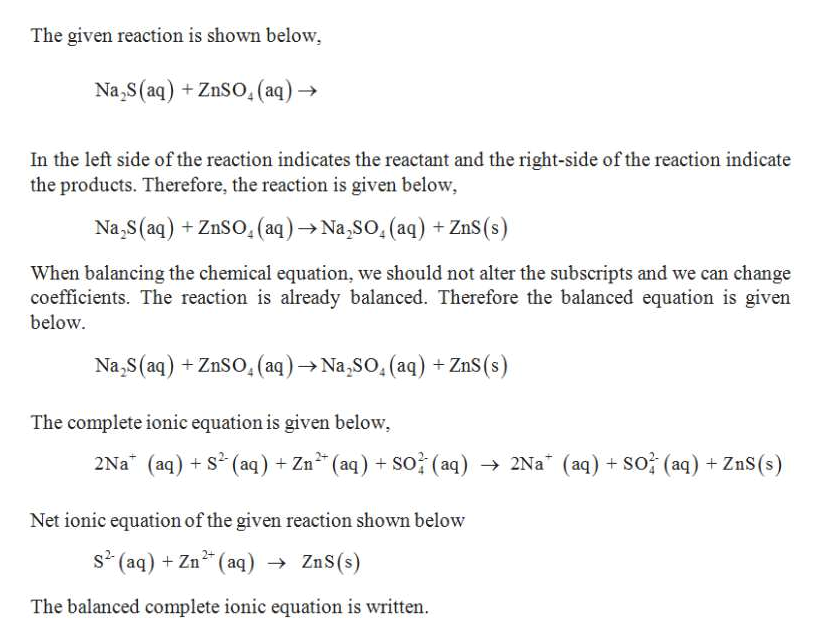# Complete the following precipitation reaction with balanced molecular, total ionic, and net ionic equations. Remember to include the proper physical states and charges of ions. When writing ions, if a charge number is not "1", place the charge number before the charge sign. If a charge number is "1", do not include the charge number in the formula. Na2S(aq) + ZnSO4(aq)→Molecular: Total ionic: Reactants Products:→ Net ionic:

Question
43 views

Complete the following precipitation reaction with balanced molecular, total ionic, and net ionic equations. Remember to include the proper physical states and charges of ions. When writing ions, if a charge number is not "1", place the charge number before the charge sign. If a charge number is "1", do not include the charge number in the formula.

Na2S(aq) + ZnSO4(aq)→

 Molecular: Total ionic: Reactants Products: → Net ionic:
check_circle

Step 1

Balancing equation:

Balancing equation in which number of atoms for each element in the reaction and the total charge are same on both reactant side and the product side.

Steps in balancing the equation,

Step...help_outlineImage TranscriptioncloseThe given reaction is shown below Na,s(aq) +ZnSO, (aq) In the left side of the reaction indicates the reactant and the right-side of the reaction indicate the products. Therefore, the reaction is given below Na,S(aq) +ZnSO, (aq)Na,So, (aq)ZnS When balancing the chemical equation, we should not alter the subscripts and we can change coefficients. The reaction is already balanced. Therefore the balanced equation is given below Na,S(aq) +ZnSO, (aq)Na,So, (aq) +ZnS(s) The complete ionic equation is given below 2Na (aq)S(aq) Zn (aq) so (aq) 2Na (aq) SO (aq) + ZnS(s) Net ionic equation of the given reaction shown below s2 (aq) Zn2 (aq) ZnS(s) The balanced complete ionic equation is written fullscreen

### Want to see the full answer?

See Solution

#### Want to see this answer and more?

Solutions are written by subject experts who are available 24/7. Questions are typically answered within 1 hour.*

See Solution
*Response times may vary by subject and question.
Tagged in

### General Chemistry